[BACK]
 Computers, Materials & ContinuaDOI:10.32604/cmc.2022.026307Article

Cost and Efficiency Analysis of Steganography in the IEEE 802.11ah IoT Protocol

1Automobile Transportation Department, South Ural State University, Chelyabinsk, 454080, Russia
2Electrical and Electronics Engineering Department Karabük University, Karabük, 78050, Turkey
3Department of Electrical Engineering, Indian Institute of Technology Ropar, Punjab, 140001, India
4Department of Computer and Network Engineering, University of Jeddah, Jeddah, 23218, Saudi Arabia
5Faculty of Engineering Technology, University Tun Hussein Onn Malaysia, Pagoh Campus, 86400, Malaysia
*Corresponding Author: Akram A. Almohammedi. Email: akrama2810@gmail.com
Received: 21 December 2021; Accepted: 22 February 2022

Abstract: The widespread use of the Internet of Things (IoT) applications has enormously increased the danger level of data leakage and theft in IoT as data transmission occurs through a public channel. As a result, the security of the IoT has become a serious challenge in the field of information security. Steganography on the network is a critical tool for preventing the leakage of private information and enabling secure and encrypted communication. The primary purpose of steganography is to conceal sensitive information in any form of media such as audio, video, text, or photos, and securely transfer it through wireless networks. In this paper, we analyse the performance characteristics of one of the steganography techniques called Hidden Communication System for Corrupted Networks (HCCNETs) for hiding sensitive data. This performance analysis includes the efficiency and the cost of the system in Wireless Local Area Networks (WLANs), specifically in the IEEE 802.11ah IoT protocol. The analysis is mainly based on a two-dimensional Markov chain model in the presence of an error channel. Additionally, the model considers packet arrival rate, back-off timer freezing, back-off stages, and short retry limit to ensure compliance with IEEE 802.11ah requirements. It stresses the importance of taking these elements into consideration while modeling the efficiency and cost of the steganographic channel system. These parameters often result in a high precise channel access estimation, a more accurate and efficient accuracy measurements system, efficient channel utilisation, avoidance of throughput saturation overestimation, and ensuring that no packet is served endlessly. Evaluated results demonstrate that HCCNETs is an effective approach at low cost.

Keywords: IoT; HCCNETs; steganography; markov chain model

1  Introduction

The authors in  proposed a steganography approach for IEEE 802.11 via using intentionally corrupted checksums frames to set up hidden communications. However, the authors in  assumed saturated condition and such assumption leads to unstable network. The steganography in IEEE 802.11 OFDM symbols was analysed in [13,14]. The authors in [13,14] proposed a model based on 2-D Markov chain to analyse the network throughput of steganographic method of IEEE 802.11 a/g standards within a non-ideal channel. Nevertheless, the authors in [13,14] considered saturated situations and analysed it within basic access method. In , the authors presented and described the elliptic Galois cryptography scheme. A cryptography approach was employed in this study to secure private data obtained from a variety of medical sources. Following that the encrypted data was embedded into a low complexity picture using a Matrix XOR encoding steganography approach. Additionally, the suggested work in  used an optimization approach called Adaptive Firefly to improve the image’s cover block selection. In , the authors described a method for concealing secret messages by mapping numerous steganographic methods to complicated texture objects. To begin, complicated texture patches are chosen using an object recognition technique. Second, three distinct steganographic techniques were utilised to conceal a hidden message inside the block area chosen. The authors in  developed a novel approach for detecting steganography in network protocols. The technique was developed using machine learning algorithms and was based on a multilayer approach for the selective examination of derived and aggregated metrics. The primary purpose was to enable steganalysis on networks with a high density of devices and connections. The authors in  introduced a large-capacity secure authenticated quantum video steganography scheme. This approach allows for the embedding of secret quantum information into carrier quantum video, significantly increasing the embedding capacity. Additionally, it accomplishes quantum information steganography via the use of video’s unique properties, as well as an authentication system for increased security. In , the authors presented CloudSteg, a steganographic technique that establishes a covert channel between two cloud instances that share a physical computer through hard disc contention. In , the authors offered a coverless information concealing approach in which original pictures with traits capable of expressing hidden information are employed directly as stegoimages. Additionally, the authors developed a revolutionary coverless information concealment technique for images utilising Faster Region-based Convolutional Neural Networks (Faster-RCNN). The authors used Faster-RCNN to recognise and locate objects in pictures and to convey hidden information through the labels of these things. The authors of  also employed deep neural network to conceal numerous speech signals under a single cover using multiple decoders or a single conditional decoder. Three distinct networks were used in . The encoder network takes as inputs a carrier and a message and creates a combined latent network for both signals. This is subsequently passed via a carrier decoder network, which outputs the carrier embedded with the message. Finally, the message decoder network reconstructs the concealed message signal from the embedded signal. They discovered that the decoded signals are indistinguishable, however this approach has the disadvantage of not operating in other audio domains outside speech.

The work presented in this paper focuses on one of steganography techniques, called the Hidden Communication System for Corrupted Networks (HCCNETs) for hidden sensitive data. The significance of HCCNETs lies on the use of a protected communications network equipped with cryptographic techniques to offer a steganography system and suggestion of new protocol with bandwidth allocation on the basis of corrupted frames. The system’s primary innovation is the use of frames with intentionally erroneous checksums to create concealed communication. This study is an extension of the existing study in , by adding an idle state to the model to reflect the empty queue at the MAC layer when no packet is available for transmission. The important contributions of this work are as follows: 1) An analytical model based on a two-dimensional Markov chain under unsaturated situations is developed. The primary advantages of using unsaturated circumstances in this model are that (i) real networks are predominantly non-saturated, (ii) saturated circumstances often result in an unstable network, and (iii) it allows for the consideration of inter-arriving time and burstiness in the network [22,23]. 2) An error-prone channel is modelled in this work in order to avoid overestimating saturated throughputs. The model also considers packet arrival rate, back-off counter freezing, back-off stages, and short retry limit to ensure compliance with IEEE 802.11ah IoT protocol requirements. It stresses the importance of taking these elements into consideration while modeling the efficiency and cost of the steganographic channel system. These parameters often result in a high precise channel access estimation, a more accurate and efficient accuracy measurements system, efficient channel utilisation, and ensuring that no packet is served endlessly. 3) Derivation of transmission probability, successful transmission probability, and collision probability is performed to express and compute the performance characteristics of the HICCUPS, such as the system’s throughput, efficiency and cost of WLAN usage in the network. The system usage cost (κ) is defined as the reduction of WLAN throughput caused by HICCUPS functioning in corrupted frame mode. The efficiency of a system (ε) is described as the throughput of the system in corrupt frame state.

The rest of the paper is arranged as follows: Section 2 presents the model analysis including the frame transmission probability in the corrupted frame mode as well as the data transmission time analysis. Section 3 describes the cost analysis κ. Section 4 discusses efficiency analysis ε. Section 5 concludes the paper.

2  The Model

In this section, the medium access procedure for nodes is formulated using two-dimension Markov Chain, then the system’s throughput, efficiency and cost of IEEE 802.11ah communication are derived. In Fig. 1, the Markov Chain model of the 802.11ah backoff mechanism within a Restricted Access Window (RAW) slot is shown in corrupted frame mode. This Markov Chain is adopted from  for unsaturated circumstances in the presence of error-prone channel. From the HCCNET’s WLAN viewpoint, communication is always unsuccessful due to a lack of valid checksums. Thus, steganogram transmission occurs at each stage of the backoff operation which allows us to predict the HCCNETs behaviour using the Markov chain-based model with probability of failure pf = 1, which means it always fails. An idle state is added to the model to reflect the empty queue at the MAC layer when no packet is available for transmission. The primary advantages of using unsaturated circumstances in this model are that (i) real networks are predominantly non-saturated, (ii) saturated circumstances often result in an unstable network, and (iii) it allows for the consideration of inter-arriving time and burstiness in the network [22,23]. Contention-based medium access control is used by the nodes to compete for channel access. An imperfect transmission channel is assumed in the model to avoid an overestimation of saturated throughput. The worst-case frame error rate–FER scenario is investigated, in which errors are randomly distributed in the transmission channel. Therefore, a Gaussian wireless error channel is considered, where a Bit Error Rate (BER) of the channel is given and each bit has the same probability of encountering a bit error. Additionally, the freezing of the back-off timer and packet arrival rate are considered in order to offer an accurate channel access estimation and efficiently use the channel. The model also considers back-off stages and short retry limit for packet transmission to comply with the IEEE 802.11ah standard and to guarantee that no packet remains served forever. Nodes communicate in ad hoc mode. The transmission range is shared with all nodes, and there are no hidden terminals on the network. Tab. 1 displays the important notations and variables utilised in the study for simplicity.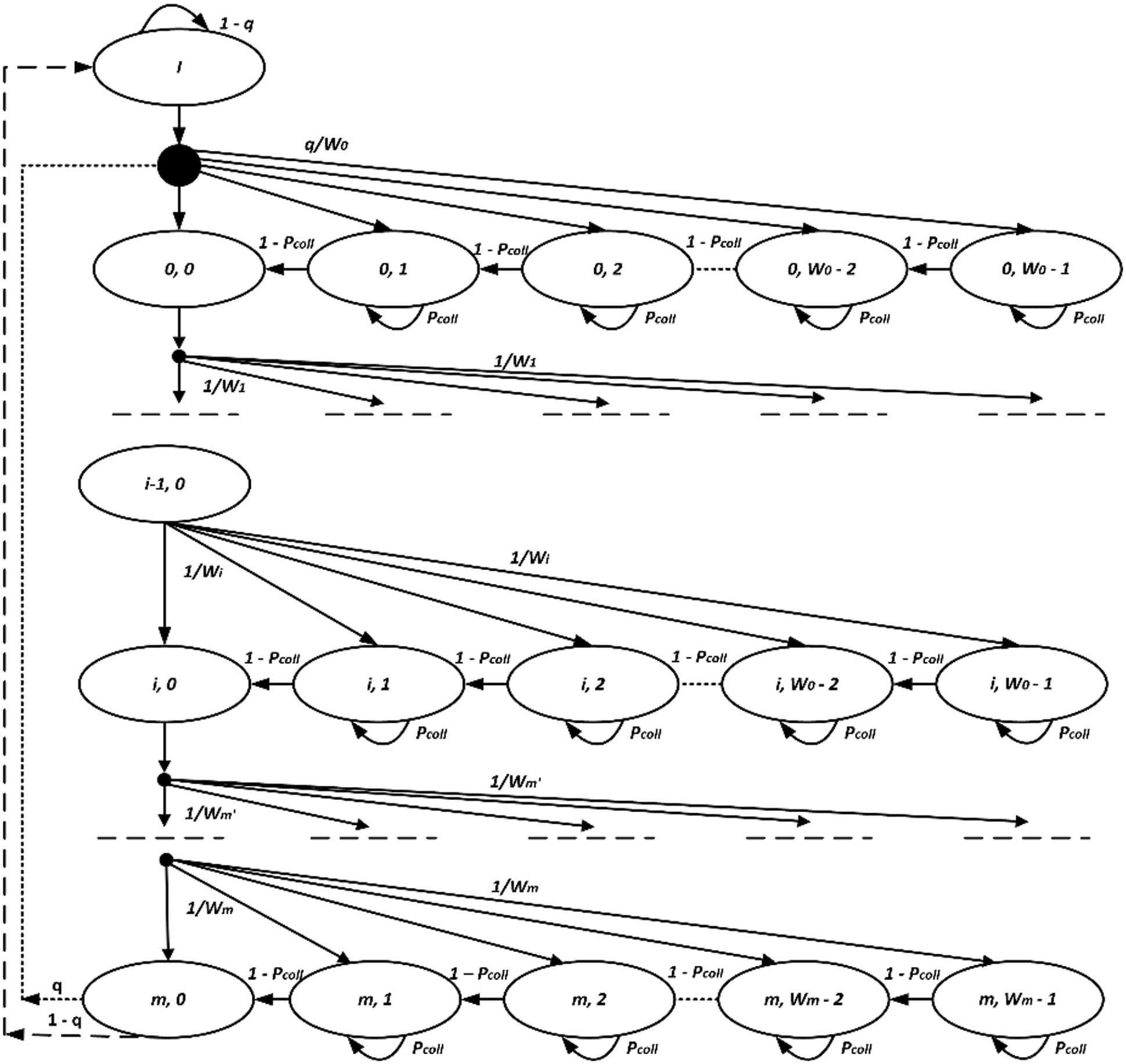Figure 1: The packets transmission process using Markov chain model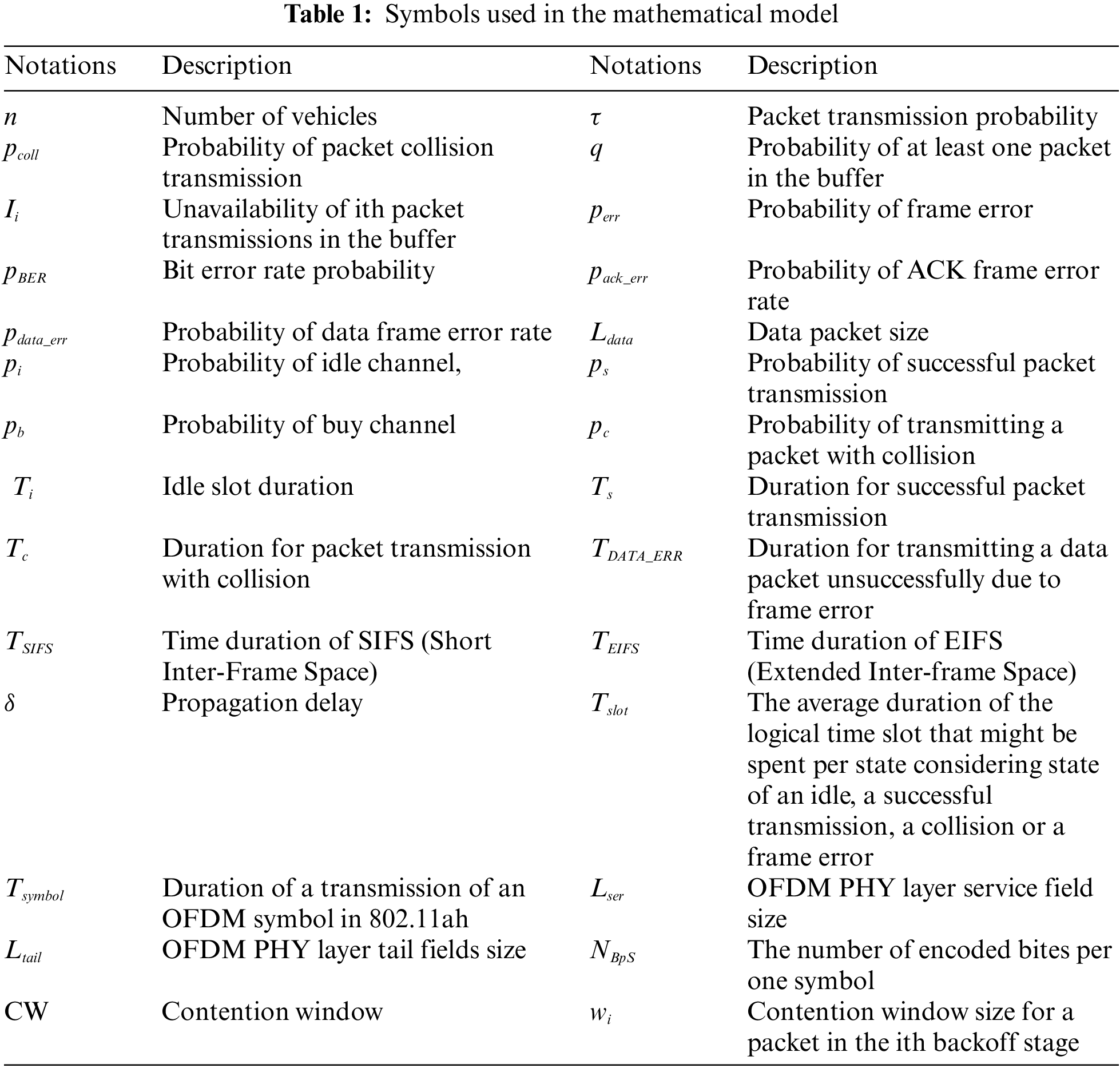2.1 Frame Transmission Probability in the Corrupted Frame Mode τcf

As seen in Fig. 1, the 2-D Markov chain model is used to determine the probability of frame transmission in the corrupted frame mode τcf. Let s(t) and b(t) be random variables denoting the back-off stages (0, 1, 2,…, m) and the value of the back-off counter (0, 1, 2,…, W i−1) for every provided station at time slot t, respectively. The highest value of the back-off counter typically depends on the back-off stages. As a result, these random variables are not self-contained.

Wi={2iW0,im2mW0,i>m(1)

W0 specifies the starting size of the contention window, W0=(CWmin+1), whereas m specifies the maximum number of times the contention window may rise based on the followings,Wm=2mW0=(CWmax+1). In this model, the m’ value is set to 5. Let m be the highest number of possible back-off stages. The two-dimensional s(t),b(t) processes, on the other hand, are assessed using a discrete-time Markov chain in which the channel state changes. Assume that the state process is denoted by (i, k). The state transition diagram of a two-dimensional Markov chain is shown in Fig. 1, and the non-zero transition probabilities are represented by Eq. (2).

{P(i,k|i,k+1)=1pcoll,0kWi2,0imP(i,k|i,k)=pcoll,1kWi1,0imP(i,k|i1,0)=1/Wi,0kWi1,1imP(0,k|m,0)=q/W0,0kW01,(2)

The first case in Eq. (2) indicates that the back-off counter reduces when the channel is detected idle. The second case in Eq. (2) indicates that when the channel is noticed busy, the back-off counter is frozen. If a packet is not successfully transmitted, the back-off phase moves from i1\; to\; i and also doubles the CW value, as shown in the third case of Eq. (2). Maximum CWs size and back-off phase values are reset to the minimum levels when the repeat limit is reached, as shown in case 4 of Eq. (2). If there is still a packet in the queue for transmission, the node commences the back-off process from the first phase.

The non-null transition probabilities in this case represent the absence of packet transmission in the buffer that is forwarded to the idle state (I) after successful transmission.

{P(I|m,0)=1qP(I|I)=1qP(0,k|I)=q/W0,0kW01(3)

Whenever the repeat limit is reached, the maximum back-off phase m and CW size value are reset to the minimum levels as shown in the first case of Eq. (3). Then, the node goes into idle mode if there are no more packets in the transmission queue. The second case in Eq. (3) denotes that the node stays in the idle mode if no new packets are received at the queue for transmission. The third case in Eq. (3) represents that the node goes from the idle mode to the back-off state k by uniformly selecting a back-off counter value in the range [0,W01].

Assume that bi,k = limtP{s(t)=i,\; b(t)=k} denotes the stationary distribution of Markov Chain, when i(0,m) and k(1,Wi1). We observe that Fig. 1 representing Markov Chain Model is different from the model in  for 802.11p Carrier-Sense Multiple Access with Collision Avoidance (CSMA/CA) in terms of back to states (0, k) for 0 ≤ k ≤ W0 − 1 and (i, 0) for 0 ≤ i ≤ m. Value one is a graphical representation of “permanently failure” from the WLAN perspective.

Due to the chain regularities, for each k (1,Wi1), the stationary distribution’s idle and back-off phases of data transmission are denoted by bI and bi,k and are expressed by Eqs. (5) and (6), respectively.

bi,k=WikWi(1pcoll){qbm,0+qbIi=0bi1,00<im(4)

bi,k=WikWi1(1pcoll)bi,0,for0im,1kWi1(5)

bI=(1q)bm,0+(1q)bI(6)

From Eq. (6), we get Eq. (7):

bI=1qqbm,0(7)

Since

i=0mbi,0=b0,0(m+1)(8)

Therefore, by applying the condition of stationary distribution normalisation,

1=i=0mk=0Wi1bi,k+bI

1=i=0mbi,0+i=0mk=1Wi1bi,k+bI(9)

We obtain Eq. (10) from Eq. (9), which is dependent on the values of m and m

b0,0={2q(1pcoll)£s,mm2q(1pcoll)¥,m>m(10)

where:

£s=(12pcoll)(m+1)q+W0(2m+11)q+2(1pcoll)(1q)(11)

And:

¥=(12pcoll)(m+1)q+W0(2m+11)q+2mW0(mm)q+2(1pcoll)(1q)(12)

Since we have b0,0, we are now able to determine the probability of a node transmitting a frame in the corrupted frame mode τcf, where a node can send a packet in a randomly chosen time slot. The node can only send a packet whenever the back-off time counter is zero (bi,0), regardless of the back-off stage, as in Eq. (13).

τcf=i=0mbi,0=b0,0(m+1)(13)

Eq. (13) demonstrates that the τcf value is dependent on the conditional collision probability pcoll and the probability of at least one packet being available in buffer q. There is a chance of a collision occurring, when at least two nodes send packets in the same time slot.

During transmission, the error frame probability perr is given by:

perr=1(1pdata_err)(14)

where pdata_err is the Frame Error Rate (FERs) for DATA frame that has been corrupted. This error probability is determined by computing the bit error probability (i.e., BER) pBER by Eq. (15):

pdata_err=1(1pBER)Ldata(15)

where the bit error rate (pBER) could be calculated by dividing the bit energy by the noise. In this study we use QPSK modulation, then the pBER for QPSK modulation could be calculated as follows by Eq. (16)

pBER=Q(2EbNo)(16)

where EbNo indicates the received signal’s bit-energy-to-noise ratio and the Q-function is defined as in Eq. (17):

Q(x)=x12πet22dt(17)

The probability of transmitting packets colliding is defined as follows:

pcoll=1(1τcf)n1(18)

We can calculate the packet transmission from Eqs. (13) and (18) by numerically solving the unknown variable τcf.

2.2 Data Transmission Time Analysis

In this subsection, the system throughput of HCCNETs in the corrupted frame mode (Scf) is analysed. Fig. 2 depicts the four channel states that may happen during the corrupted frame manner. In this mode, all 802.11ah packets contain an intentionally erroneous CRC-32 code value entered into the Frame Checksum Control (FCS) field. As a result, there are no ACKnowledgement (ACK) frames used to provide positive feedback, and thereby the ACK error status is neglected. Successful transmission in the HCCNETs, which is not defined in the same manner as in the 802.11ah network, indicates that there are no collisions or data errors during transmission. The HICCUPS frame integrity mechanism is separated from the 802.11ah FCS. Throughout the contention-based MAC method, the channel state will be one of the following during each time slot: idle, successful transmission, collision transmission, or failure transmission due to frame error. As a consequence, the channel state probability is expressed as Eq. (19).

{pi=(1τcf)nps=nτ(1τcf)n1(1pdata_err)pc=1(1τcf)nnτs(1τcf)n1pDATA_ERR=nτ(1τcf)n1pdata_err(19)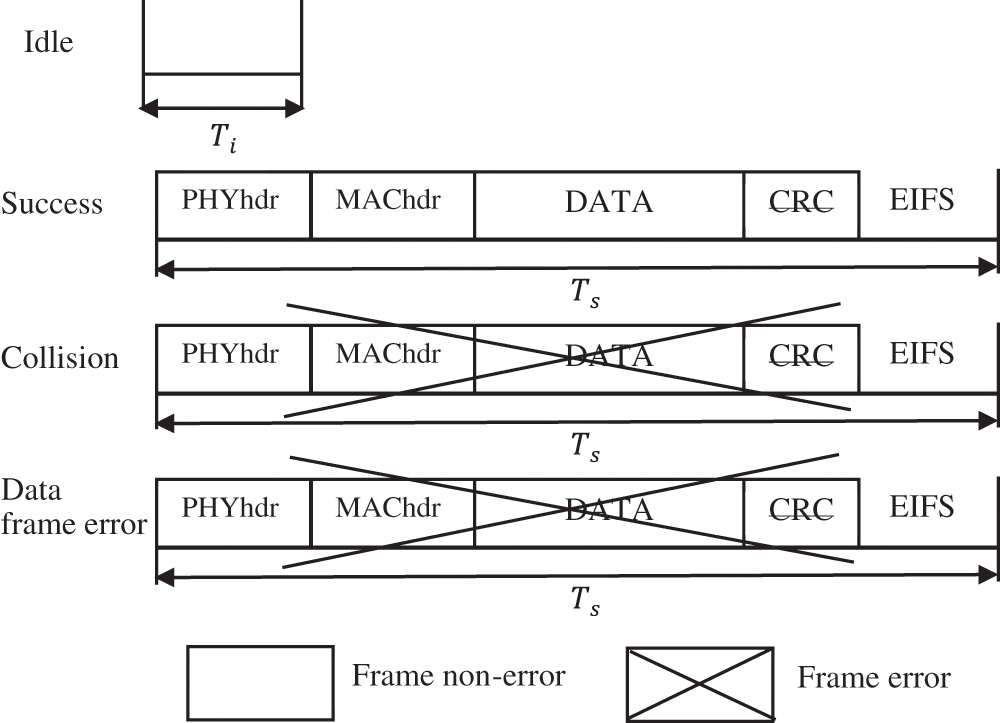Figure 2: Time slots length for the packet process of transmission

Fig. 2 illustrates the time slot lengths for the packet process of transmission using the contention-based MAC for DATA frames. Thus, as in Eq. (20), the transmission time is calculated using the unicast mode.

{Ti=TσTs=Th+Tdata+δ+TDIFSTc=TsTDATA_ERR=TsTACK_ERR=Ts(20)

where Tdata=Lpld/R, Lpld indicates the payload of the data frame with FCS field and R is the data transmission rate. Tdata is PHY-layer dependent and the transmission of a frame in terms of Orthogonal Frequency-Division Multiplexing (OFDM) symbols is represented as Eq. (21):

Tdata=TsymbolLser+Ltail+LdataNBpS(21)

Thus, the duration of the logical time slots Tslot 802.11ah per state on the channel is computed by Eq. (22) in order to calculate the network throughput, which is defined as follows:

Tslot=piTi+psTs+pcTc+pDATA_ERRTDATA_ERR(22)

Eventually, the system throughput of HCCNETs in the corrupted frame mode (Scf) is expressed by Eq. (23)

Scf=psLpldTslot(23)

3  Cost Analysis κ

The cost κ, as defined in the first section of this study, is the difference between S, in the presence of frame error rate without HCCNETs, and S, with frame error rate resulting from HCCNETs in the corrupted frame manner. Simply, it is a drop of WLAN throughput caused by HCCNETs hidden channels.

Assume that frame error rate increases with the fixed value △FER when applying HCCNETs as shown in Fig. 3. The frame error rate for networks without HCCNETs is equal to FER’. It can be seen that △FER ≤ 1 – FER. Thus, we may represent the cost as follows:

κ=S(FER)S(FER+FER)(24)

Then, it is normalised to R as:

κ¯=κR(25)

Since the cost curves are derived on S(FER) and appear to be nearly linear, so, we may apply the following approximation formula for small values of △FER, as shown in Fig. 4:

κFER1FERS(FER)(26)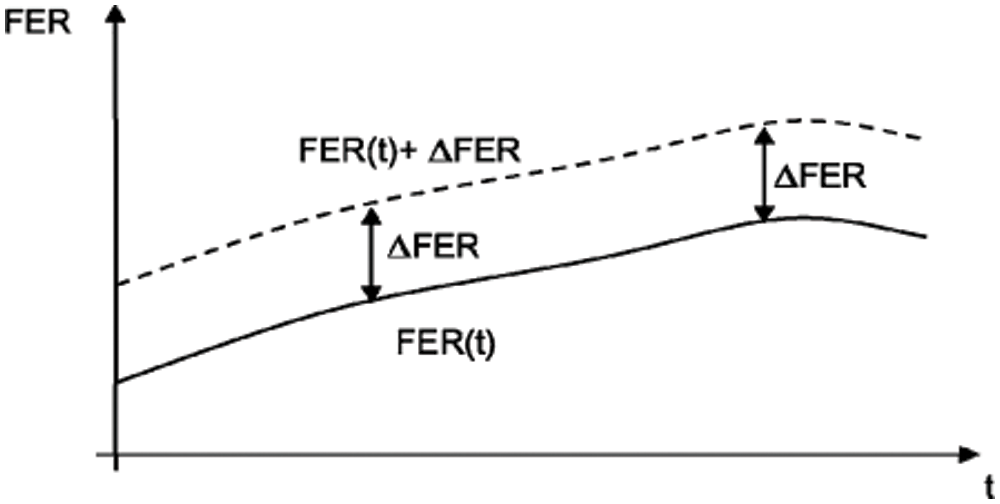Figure 3: Interpretation of ΔFER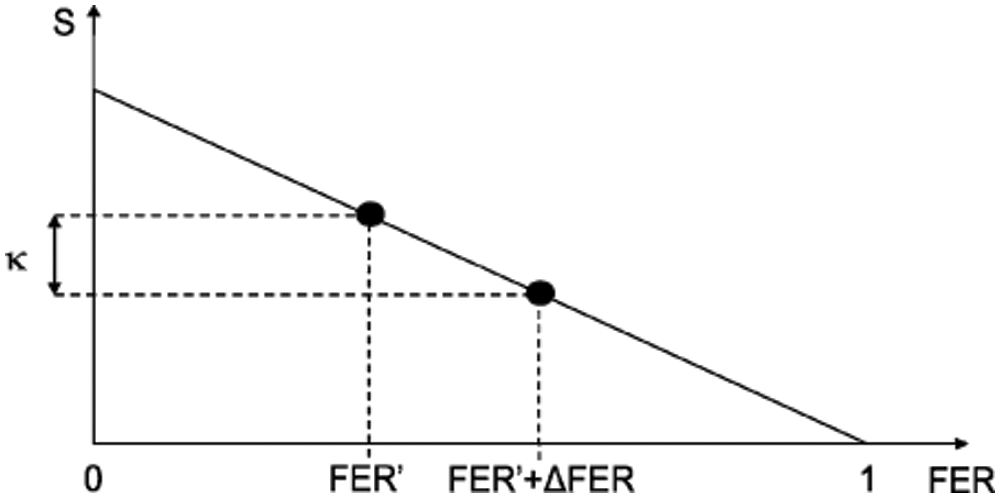Figure 4: Illustration of the cost κ 

The cost values for IEEE 802.11ah (ERP-OFDM) are shown in Tabs. 2 and 3, when n = 10, 20, and R = 6.5 Mbps. These values, when L = 1000 bytes, are obtained from Eq. (26), and are computed for FER ∈ {0; 0.0769; 0.5507}. These FER values correspond to the following three BER = 0, 105, 104. Five typical values of △FER are considered under these scenarios (0.01; 0.02; 0.03; 0.04; 0.05).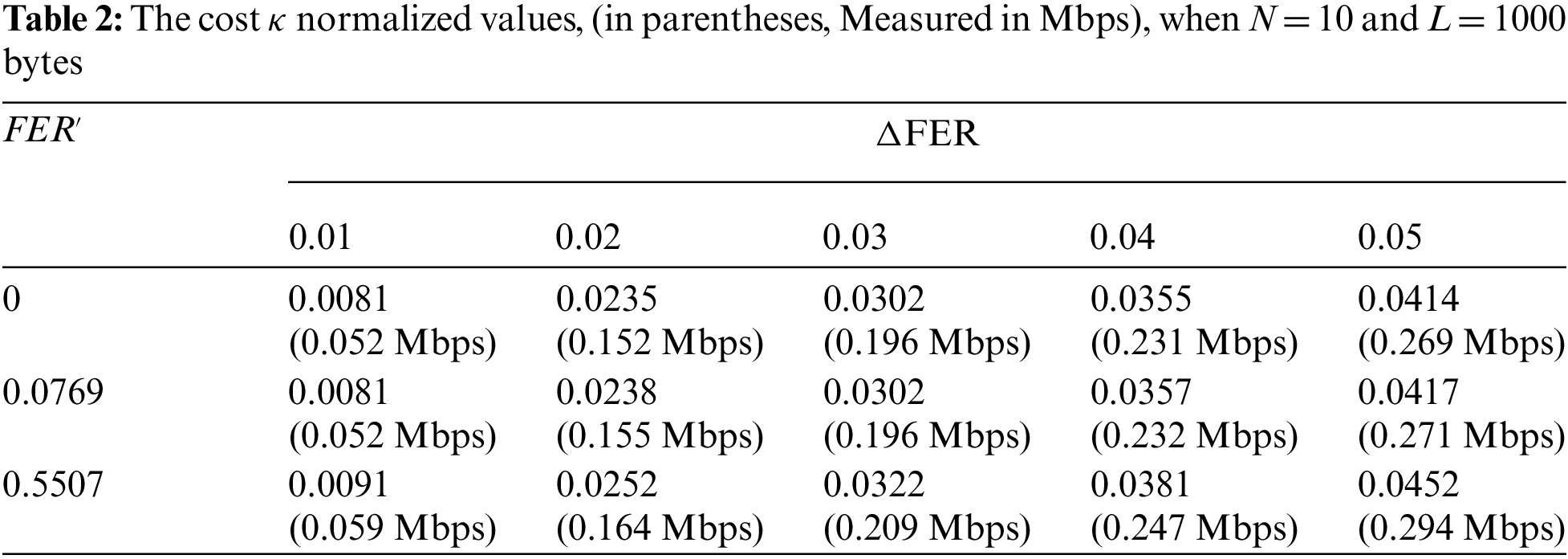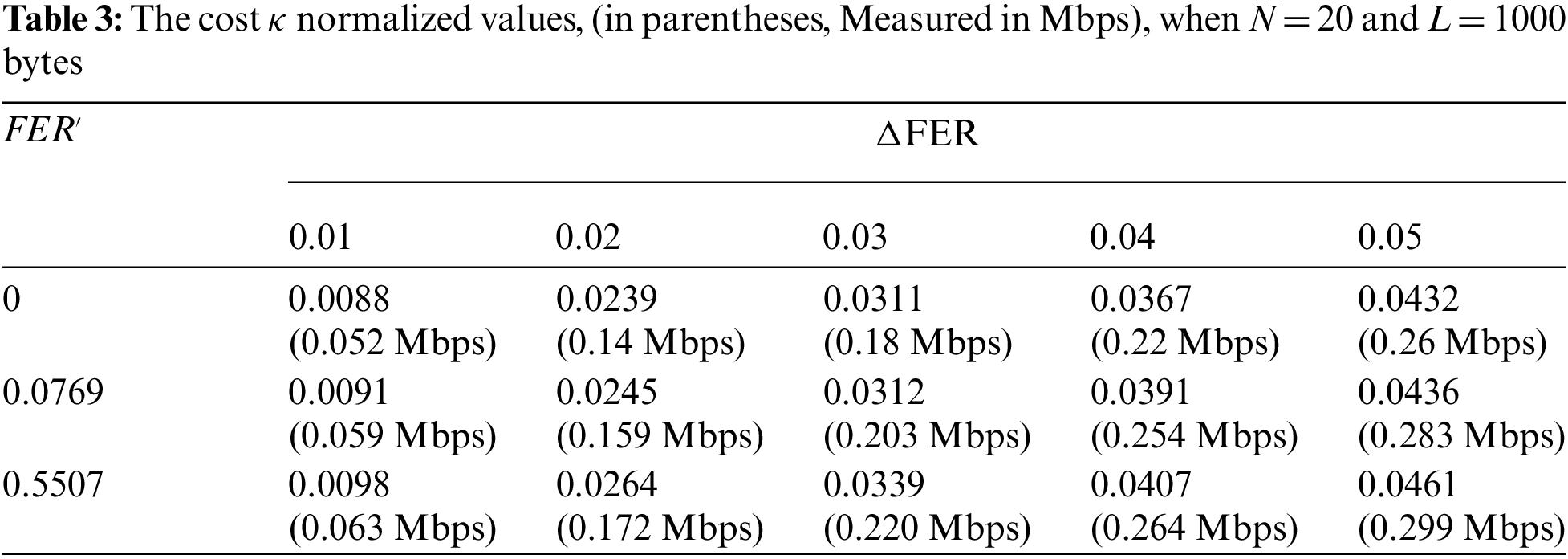4  Efficiency Analysis ε

The efficiency is defined as the Scf in situations caused by the physical channel (particularly BER) and the number of frames consumed by the HCCNETs in the corrupted frame manner. These situations provide a different view of FER from the perspective of the HCCNETs such as the appropriate frames in the HCCNETs are bad for a WLAN, and certainly the ideal frames for a WLAN in the presence of the HCCNETs are considered incorrect. As a result, we will use FERcf to emphasise this distinction and express ε as follows:

ε=Scf(FERcf)(27)

Scf, analysed in the previous section of the work, is used to compute the upper limit of the system throughput for HCCNETs. Corrupted frame mode happens seldom during typical operation of the HCCNETs. Two scenarios are used to validate the efficiency. In the first scenario, all stations operate exclusively in damaged frame manner (the HCCNETs is on at all times).

Since S(1) equals 0, then S = 0 in the FER function; and Scf=Scf(FER) in the FER function. Since 0 ≤ △FER ≤ 1 − FER, △FER = 1 − FER. The HCCNETs is off at all times in the second scenario (△FER = 0, just typical transmission is executed,

Therefore Scf = 0 (since Scf(1) equals 0), S is equal to S(FER). Based on the two scenarios discussed previously, we can evaluate the hypothetic point of operation of HCCNETs for (FER + △FER) as a mix of reflection and translation, as shown in Fig. 5. The Scf curve is reflected and then translated into the FER domain in order to maintain the relationship between S(1) = 0 and Scf(FER), as well as between S(FER) and Scf(1) = 0. Following these procedures, we can see that FERcf = 1−△FER.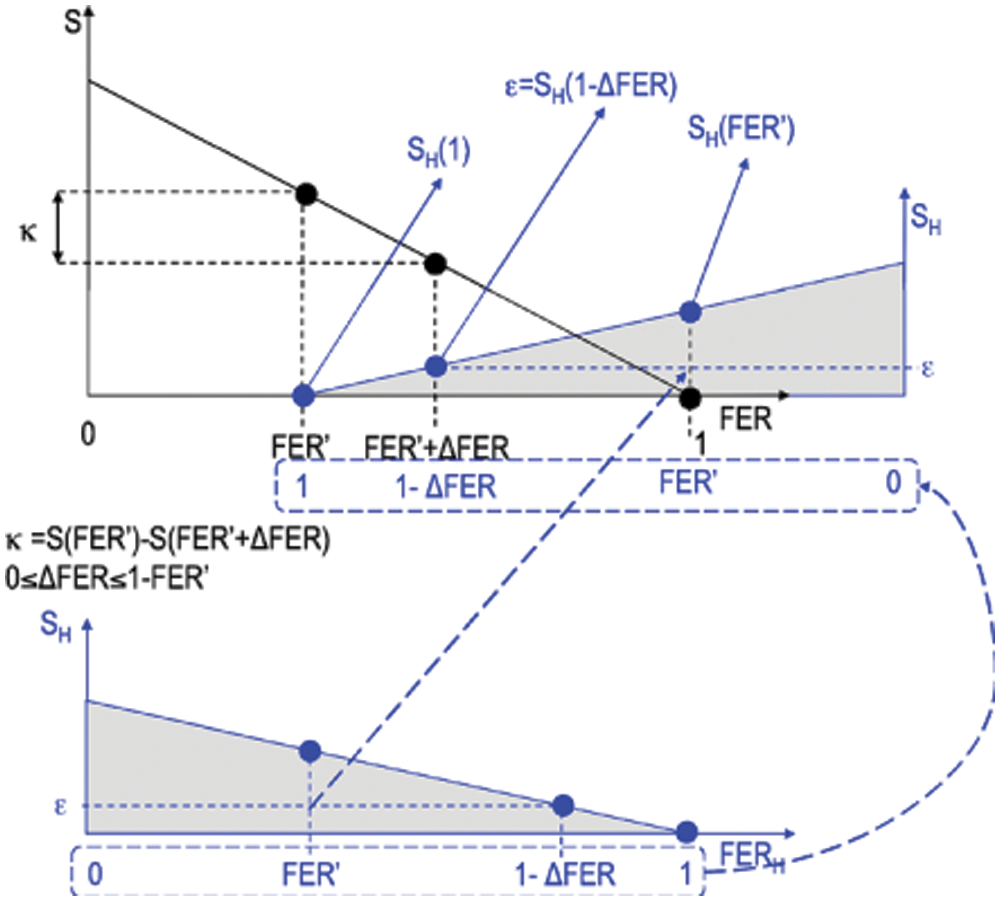Figure 5: The efficiency ε illustration 

Eventually ε is given by Eq. (28) as follows:

ε=Scf(1FER)(28)

And then normalize it to R as follows:

ε¯=εR(29)

As cost analysis, we analyse an IEEE 802.11ah (ERP-OFDM) when R = 6.5 Mbps, L = 1000 bytes frames, n = 10, 20, and the same values of △FER (0.01; 0.02; 0.03; 0.04; 0.05). Tab. 4 shows the findings of the experiment.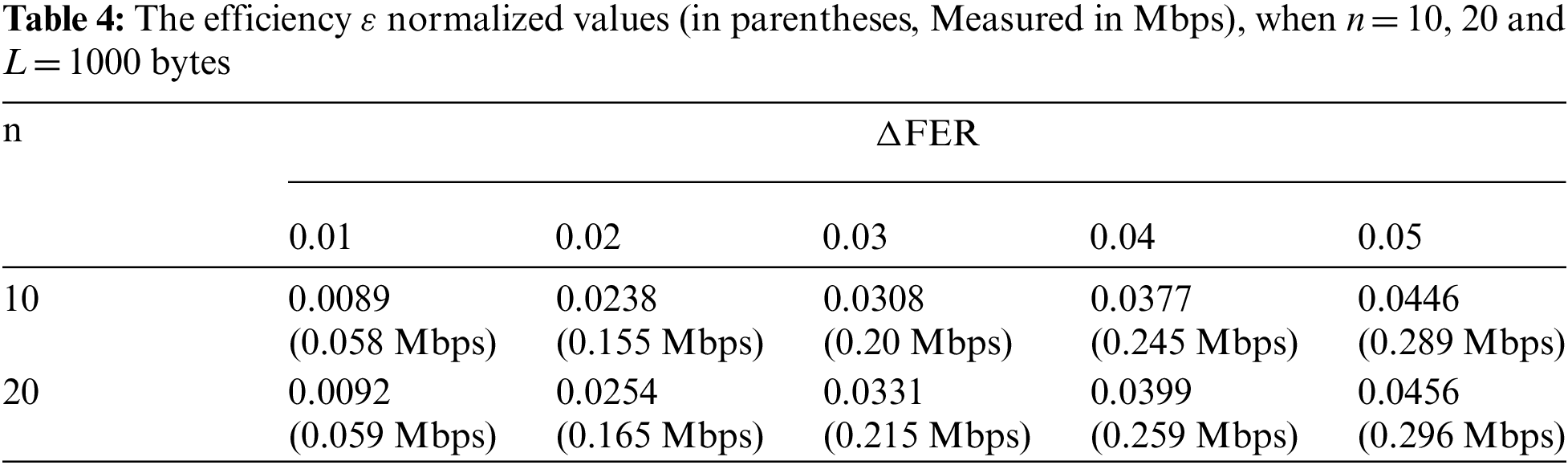The cost relies on the frame error rate, while the efficiency relies only on the △FER. For instance, in IEEE 802.11ah (ERP-OFDM), when n= 10 stations, and △FER= 0.05 with R = 6.5 Mbps, the efficiency ε = 0.289 Mbps and the cost ε = 0.294 Mbps. Moreover, when n = 20 stations and △FER= 0.05 with R = 6.5 Mbps, the efficiency ε = 0.296 Mbps and the cost κ = 0.299 Mbps. The work shows that HICCUPS is a significant method as it results in a reasonable cost and highly efficient steganographic technology.

5  Conclusion and Future Work

This paper introduces one of the steganographic techniques called HCCNETs to evaluate the performance efficiency and the cost of system usage of the steganographic channel over IEEE 802.11ah IoT protocol. We begin by analysing the IEEE 802.11ah protocol using a two-dimensional Markov chain model under unsaturated situations with an imperfect transmission channel. The analysis of the 802.11ah IoT protocol is used to determine the transmission probability, successful transmission probability, and collision probability. Then, using these derivatives formulas, performance metrics for throughput, efficiency, and cost of system usage in the network are expressed and calculated. The influence of the channel conditions and node number is examined in order to evaluate and understand the efficiency and the cost of system usage in HICCUPS over 802.11ah IoT protocol. The analytical findings indicate that HICCUPS steganographic technique is significantly efficient with reasonable cost.

Future study will concentrate on simulation analysis of HCCNETs over IoT scheme in order to analyse HCCNETs characteristics in a variety of situations and to provide a comprehensive evaluation of the security of HCCNETs.

Funding Statement: The authors received no specific funding for this study.

Conflicts of Interest: The authors declare that they have no conflicts of interest to report regarding the present study.

References

1.  1.  R. Meng, Q. Cui, Z. Zhou, Z. Fu and X. Sun, “A steganography algorithm based on cyclegan for covert communication in the internet of things,” IEEE Access, vol. 7, pp. 90574–90584, 2019.
2.  2.  S. Doss, J. Paranthaman, S. Gopalakrishnan, A. Duraisamy, S. Pal et al., “Memetic optimization with cryptographic encryption for secure medical data transmission in IoT-based distributed systems,” Computers, Materials & Continua, vol. 66, no. 2, pp. 1577–1594, 2021.
3.  3.  A. Kamilaris and A. Pitsillides, “Mobile phone computing and the internet of things: A survey,” IEEE Internet of Things Journal, vol. 3, no. 6, pp. 885–898, 2016.
4.  4.  T. Qiu, R. Qiao and D. O. Wu, “EABS: An event-aware backpressure scheduling scheme for emergency internet of things,” IEEE Transactions on Mobile Computing, vol. 17, no. 1, pp. 72–84, 2017.
5.  5.  I. Qadeer and M. K. Ehsan, “Improved channel reciprocity for secure communication in next generation wireless systems,” Computers, Materials & Continua, vol. 67, no. 2, pp. 2619–2630, 2021.
6.  6.  T. Qiu, X. Wang, C. Chen, M. Atiquzzaman and L. Liu, “TMED: A spider-web-like transmission mechanism for emergency data in vehicular ad hoc networks,” IEEE Transactions on Vehicular Technology, vol. 67, no. 9, pp. 8682–8694, 2018.
7.  7.  Y. Xu, L. Qi, W. Dou and J. Yu, “Privacy-preserving and scalable service recommendation based on simhash in a distributed cloud environment,” Complexity, vol. 2017, pp. 9, 2017.
8.  8.  L. Qi, R. Wang, C. Hu, S. Li, Q. He et al., “Time-aware distributed service recommendation with privacy-preservation,” Information Sciences, vol. 480, pp. 354–364, 2019.
9.  9.  C. Hu, W. Li, X. Cheng, J. Yu, S. Wang et al., “A secure and verifiable access control scheme for big data storage in clouds,” IEEE Transactions on Big Data, vol. 4, no. 3, pp. 341–355, 2017.
10. 10. L. Qi, S. Meng, X. Zhang, R. Wang, X. Xu et al., “An exception handling approach for privacy-preserving service recommendation failure in a cloud environment,” Sensors, vol. 18, no. 7, pp. 2037, 2018.
11. 11. A. A. Abd El-Latif, B. Abd-El-Atty, M. S. Hossain, S. Elmougy and A. Ghoneim, “Secure quantum steganography protocol for fog cloud internet of things,” IEEE Access, vol. 6, pp. 10332–10340, 2018.
12. 12. K. Szczypiorski, “A performance analysis of HICCUPS—A steganographic system for WLAN,” Telecommunication Systems, vol. 49, no. 2, pp. 255–259, 2012.
13. 13. K. Szczypiorski and W. Mazurczyk, “Steganography in IEEE 802.11 OFDM symbols,” Security and Communication Networks, vol. 9, no. 2, pp. 118–129, 2016.
14. 14. K. Szczypiorski and W. Mazurczyk, “Hiding data in OFDM symbols of IEEE 802.11 networks,” in 2010 Int. Conf. on Multimedia Information Networking and Security, pp. 835–840, Nanjing, China, IEEE, 2010.
15. 15. M. Khari, A. K. Garg, A. H. Gandomi, R. Gupta, R. Patan et al., “Securing data in internet of things (IoT) using cryptography and steganography techniques,” IEEE Transactions on Systems, Man, and Cybernetics: Systems, vol. 50, no. 1, pp. 73–80, 2019.
16. 16. R. Meng, S. G. Rice, J. Wang and X. Sun, “A fusion steganographic algorithm based on faster R-CNN,” Computers, Materials & Continua, vol. 55, no. 1, pp. 1–16, 2018.
17. 17. M. Smolarczyk, K. Szczypiorski and J. Pawluk, “Multilayer detection of network steganography,” Electronics, vol. 9, no. 12, pp. 2128, 2020.
18. 18. S. Chen and Z. Qu, “Novel quantum video steganography and authentication protocol with large payload,” International Journal of Theoretical Physics, vol. 57, no. 12, pp. 3689–3701, 2018.
19. 19. B. Lipinski, W. Mazurczyk and K. Szczypiorski, “Improving hard disk contention-based covert channel in cloud computing,” in 2014 IEEE Security and Privacy Workshops, pp. 100–107, San Jose, CA, USA, IEEE, 2014.
20. 20. Z. Zhou, Y. Cao, M. Wang, E. Fan and Q. Wu, “Faster-RCNN based robust coverless information hiding system in cloud environment,” IEEE Access, vol. 7, pp. 179891–179897, 2019.
21. 21. P. Praveenkumar, M. Nagadinesh, P. Lakshmi, K. Thenmozhi, J. B. Rayappan et al., “Convolution & viterbi EN (DE) coders on OFDM hides, rides & conveys message—A neural STEGO,” in 2013 Int. Conf. on Computer Communication and Informatics, pp. 1–5, Coimbatore, India, IEEE, 2013.
22. 22. C. Song, G. Tan, C. Yu, N. Ding and F. Zhang, “APDM: An adaptive multi-priority distributed multichannel MAC protocol for vehicular ad hoc networks in unsaturated conditions,” Computer Communications, vol. 104, pp. 119–133, 2017.
23. 23. A. A. Almohammedi and V. Shepelev, “Saturation throughput analysis of steganography in the IEEE 802.11 p protocol in the presence of non-ideal transmission channel,” IEEE Access, vol. 9, pp. 14459–14469, 2021.
24. 24. A. A. Almohammedi, N. K. Noordin, A. Sali, F. Hashim, W. A. Jabbar et al., “Modeling and analysis of IEEE 1609.4 MAC in the presence of error-prone channels,” International Journal of Electrical & Computer Engineering, vol. 9, no. 5, pp. 3531–3541, 2019.
25. 25. A. A. Almohammedi, N. K. Noordin, A. Sali, F. Hashim and S. Saeed, “A comprehensive performance analysis of IEEE 802.11 p based MAC for vehicular communications under non-saturated conditions,” Journal of ICT Research & Applications, vol. 11, no. 1, pp. 92–113, 2017.This work is licensed under a Creative Commons Attribution 4.0 International License, which permits unrestricted use, distribution, and reproduction in any medium, provided the original work is properly cited.